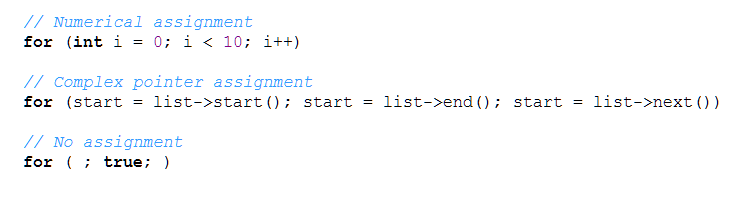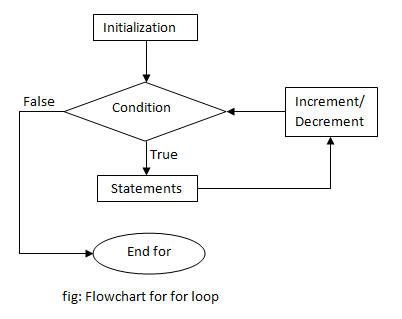# for loop in C++ Programming

In C++ programming, loop is a process of repeating a group of statements until a certain condition is satisfied. Like while loop, for loop is an entry controlled loop, where entry controlled means the condition is checked at the beginning of loop. For loop is suitable to use when the number of times a loop runs is known or fixed.

### Syntax of for loop

```for (initialization; condition; increment/decrement)
{
statement(s);
... ... ...
}```

### Components of for loop

For loop consists of three components

• Initialization
This is a part where a variable is initialized for the loop. It can be a simple numerical assignment or a complex pointer to the start of a list array. However, it is not mandatory to assign a variable. Loops without initialization would only have a semicolon “;“.
For example:• Condition
Here, condition for executing the loop is checked. It is evaluated on each loop and runs until the condition is satisfied, otherwise the control exits the loop. This is the only mandatory part of for loop.
• Increment/Decrement
This part increments or decrements the value of a variable that is being checked. The control of a program shifts to this part at the end of each loop and doesn’t necessarily have to be an increment/decrement statement as shown by the above diagram (Complex pointer assignment). It is also not mandatory to have any statement here as shown by the above diagram (No assignment).

### Flowchart of for loop### Example of for loop

C++ program to count the number of vowels in a word.

```#include <iostream>
#include <conio.h>
#include <cstring>>
using namespace std;

int main()
{
int i,vowel=0;
char word;
cout <<"Enter a word"<<endl;
cin >> word;
for (i=0;i<strlen(word);i++)
{
if (word[i]=='a' || word[i]=='e' || word[i]=='i' || word[i]=='o' || word[i]=='u')
vowel++;
}
cout <<"Total vowels = "<<vowel;
getch();
return 0;
}
```

This program counts the number of vowels in a word entered by user using for loop. For this, we need to get the number of letters in a word and loop through them to check if they are vowels or not. Since, it is easy to know the length of the input string, for loop is suitable. Every time a vowel is encountered, the value of vowel, which is initially zero is incremented. After the loop exits, the total number of vowels is known, which is printed.

Output

```Enter a word
beautiful
Total vowels = 5```